# Introduction

ARIMA (autoregressive integrated moving average) is a commonly used technique utilized to fit time series data and forecasting. It is a generalized version of ARMA (autoregressive moving average) process, where the ARMA process is applied for a differenced version of the data rather than original.

Three numbers $$p$$, $$d$$ and $$q$$ specify ARIMA model and the ARIMA model is said to be of order $$(p,d,q)$$. Here $$p$$, $$d$$ and $$q$$ are the orders of AR part, Difference and the MA part respectively.

AR and MA- both are different techniques to fot stationary time series data. ARMA (and ARIMA) is a combination of these two methods for better fit of the model.

In this write up an overview of AR and MA process will be given. The steps of building an ARIMA model will be explained. Finally, a demostration using R will be presented.

# Auto Regression (AR) and Moving Average (MA) process

## Auto Regression (AR)

AR is a class of linear model where the varible of interest is regressed on its own lagged values. If $$y_t$$ is modeled via AR process, it is written as

$y_t=\delta+\phi_1y_{t-1}+\phi_2y_{t-2}+\dots + \phi_py_{t-p}+ \epsilon_t$

The above has a form similar to simple linear regression. It has an intercept like term ($$\delta$$), regressors ($$y_{t-i}$$), parameters ($$\phi_{t-i}$$) and an error term($$\epsilon$$). The only special thing is the regressors are the dependent variable’s own lagged terms. If lag up to $$p$$ is included in the model like above, the AR process is said to be of order $$p$$.

## Moving Average (MA)

MA is another class of linear model. In MA, the output or the variable of interest is modeled via its own imperfectly predicted values of current and previous times. It can be written in terms of error terms:

$y_t=\mu+\theta_1\epsilon_{t-1}+\theta_2\epsilon_{t-2}+\dots + \theta_q\epsilon_{t-q}+ \epsilon_t$

Again, it has a form similar to classic linear regression. The regressors are the imperfections (errors) in predicting previous terms. Here the model is specified with positive sign for the parameters. It is not uncommon where we have negative sign for the parameters.

The model above included errors for $$q$$ lags and said to have an order of $$q$$.

# Steps to make ARIMA model for time series

If a process is $$ARIMA(p,d,q)$$ then the differenced data is $$ARMA(p,q)$$ process. The $$ARMA(p,q)$$ process has the following mathematical form:

$y_t=\delta+\{\phi_1y_{t-1}+\phi_2y_{t-2}+\dots + \phi_py_{t-p}\} + \{\theta_1\epsilon_{t-1}+\theta_2\epsilon_{t-2}+\dots + \theta_q\epsilon_{t-q}\} +\epsilon_t$

$\implies y_t=\delta+ \sum_{i=1}^{p}\phi_iy_{t-i}+ \sum_{j=1}^{q}\theta_j\epsilon_{t-j}+ \epsilon_t$

Three major steps can be followed to make an ARIMA forecast model:

• Model specification
• Parameter estimation
• Diagnostics and potential improvement

## Model specification

It involves identifying three numbers $$p,d,q$$ which specifies ARIMA model.

• First we determine the value of $$d$$. We first check the stationarity of the data. If the data is stationary, then $$d=0$$. If the data is trendy then we take the first difference and check for stationarity again. We keep taking differences until we get a stationary output. The number of difference required is the number $$d$$.

• There are different tools to detect stationarity. Visual observation of the data plot, auto correlation, variaogram are the most common.
• After determining $$d$$, we can utilize sample PACF (partial auto correlation) to get the AR order $$p$$. If the PACF cuts off after some lags, that number is the order of AR.

• We can determine the MA order $$q$$ by looking at the sample ACF (auto correlation) of the differenced data. If the ACF cuts off after some lags, that number is the order of MA.

Theoretically, ACF of PACF cut off if one of the AR or MA orders is zero. If both of them are non zero, then both ACF and PACF may exhibit damped sinusoid and/or exponential decay. More works to do in these cases to indentify the model specifications.

Following figure shows the ACF and PACF plot of a simulated time series. For the series, the ACF cuts off afer lag 2 and PACF cuts off after lag 1. So three potential $$(p,q)$$ specification would be $$(1,0)$$, $$(0,2)$$ and $$(1,2)$$.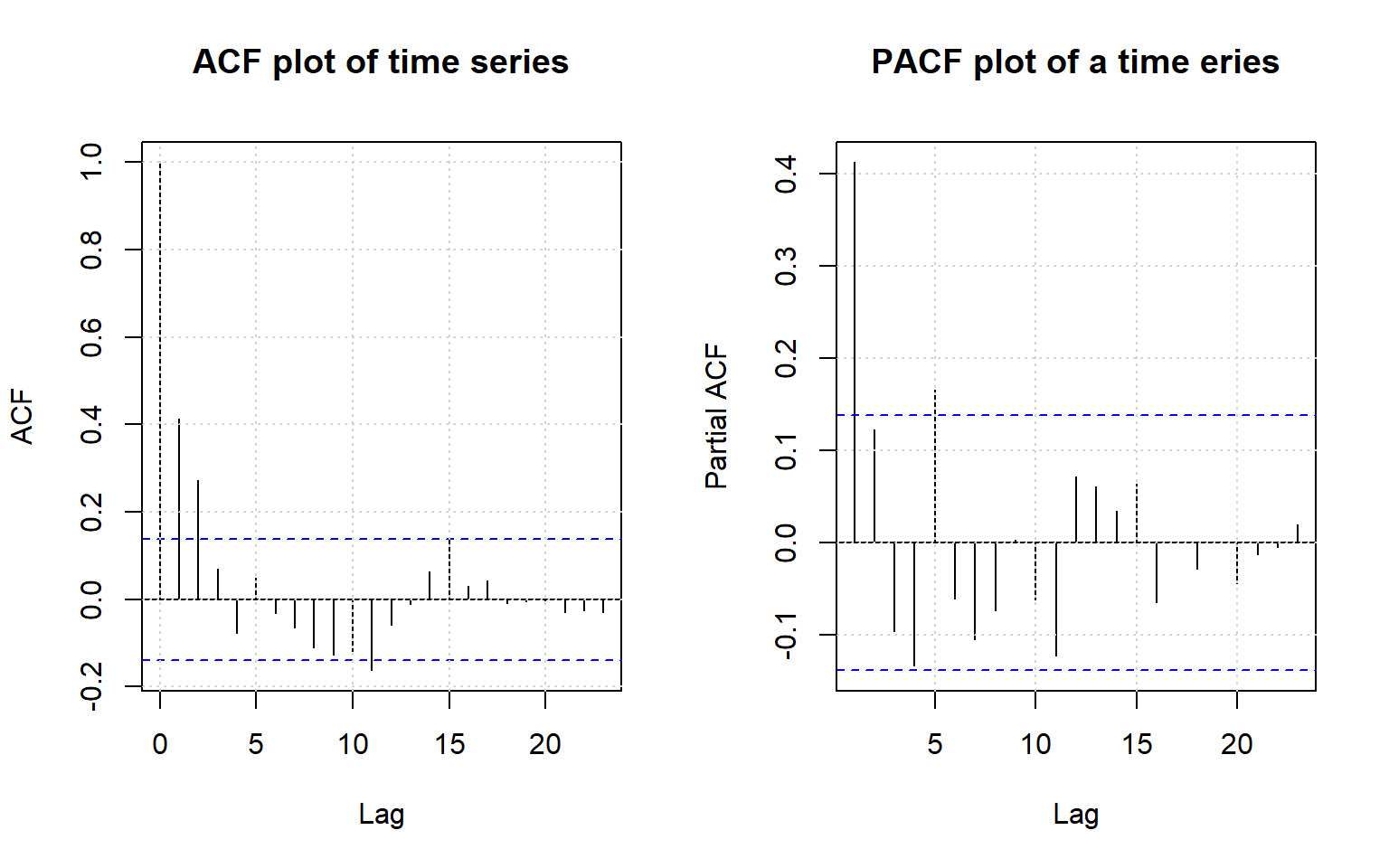## Parameter estimation

Once we get the orders, the next step is to find the parameters ($$\delta$$, $$\phi_is$$, $$\theta_js$$). Commonly used techniques are least square, maximum likelihood, method of momonts.

## Diagnostic

Once we make the model, we check the model adequacy by checking the assumptions. We mainly check the normality and aurto correlation of the residuals and look for further improvement.

# Example in R

For a demonstration purpose we will work on simulated data. We have generated 200 data points from a certain ARIMA process.

set.seed(250)
timeseries=arima.sim(list(order = c(1,1,2), ma=c(0.32,0.47), ar=0.8), n = 50)+20

## Model Specification

### Finding d

Following we plotted the original time series and the first difference. The original plot shows a clear non stationarity. the acf does not cuts off, rather it shows a slow decrease. On the other hand, the first difference data looks much stationary. The acf cuts off after some lags. We can perform other formal test to test the stationarity of the differenced data. But here from plot of the data and the acf plot provide good indication that the differenced data is stationary. Since it took one differencing to get stationarity, here $$d=1$$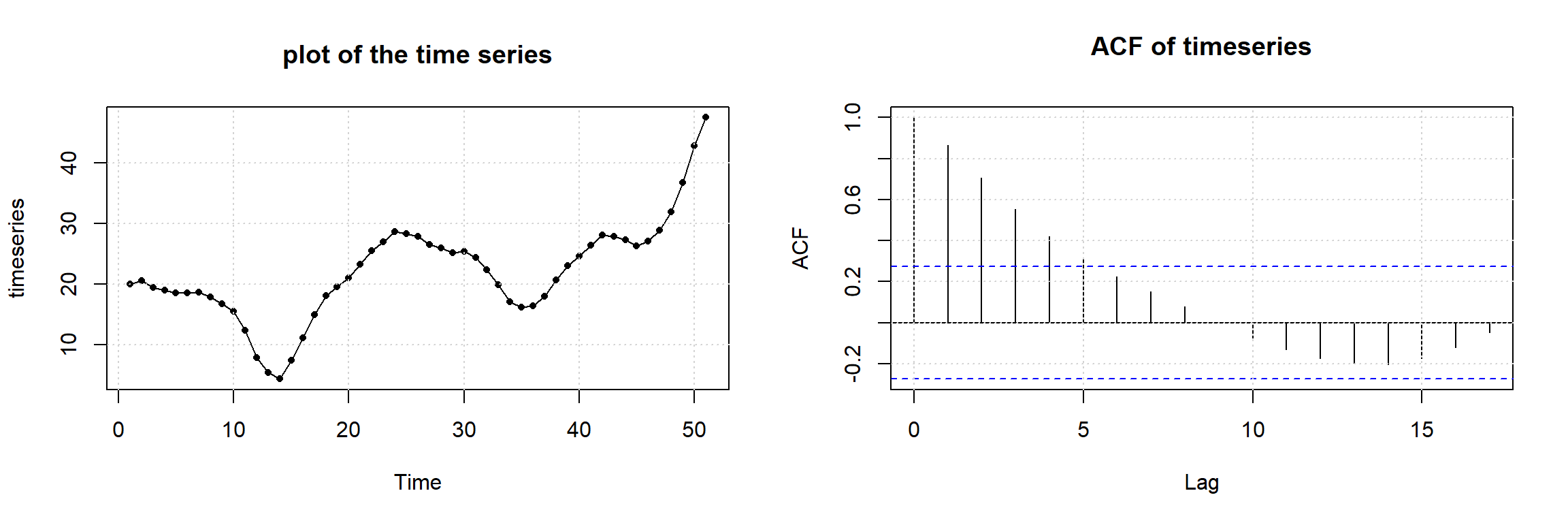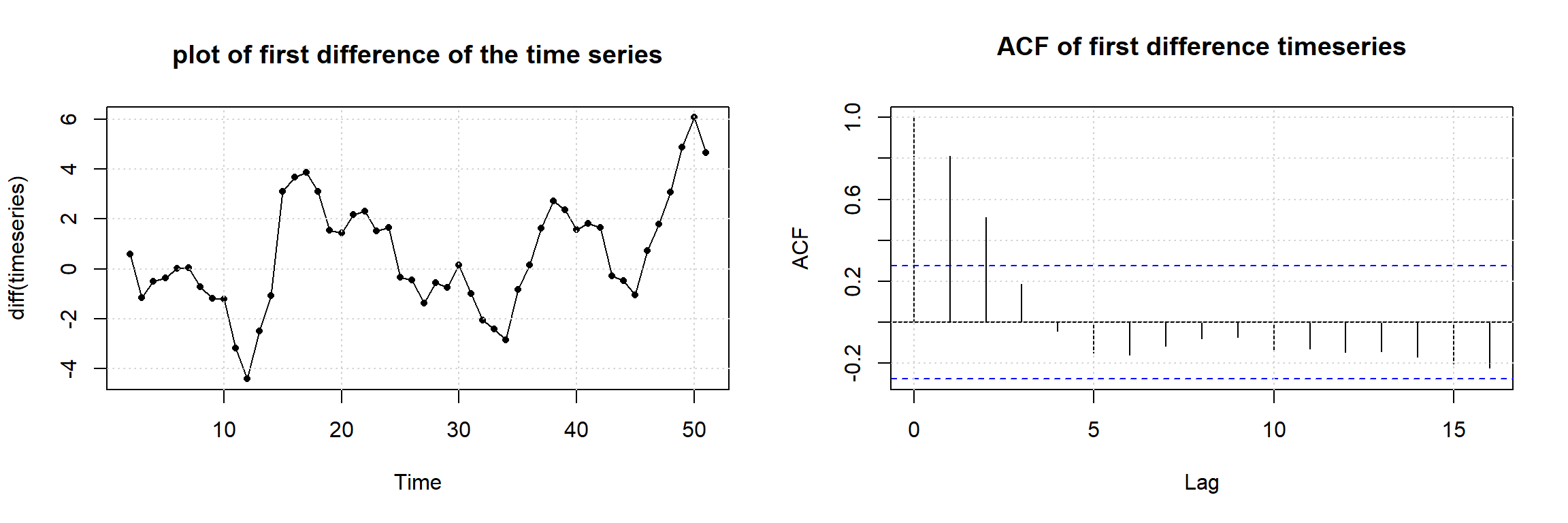### Finding p and q

The next step is to get the values of $$p$$ and $$q$$, the order of AR and MA part. To do so, we plotted the sample ACF and PACF of the first differenced data. We see that the sample ACF cuts off after lag 2 and the sample PACF cuts off after lag 1. So we propose three ARMA models for the differenced data: $$ARMA(0,2)$$, $$ARMA(1,0)$$ and $$ARMA(1,2)$$. That is, for the original time series, we propose three ARIMA models, $$ARIMA(0,1,2)$$, $$ARIMA(1,1,0)$$ and $$ARMA(1,1,2)$$.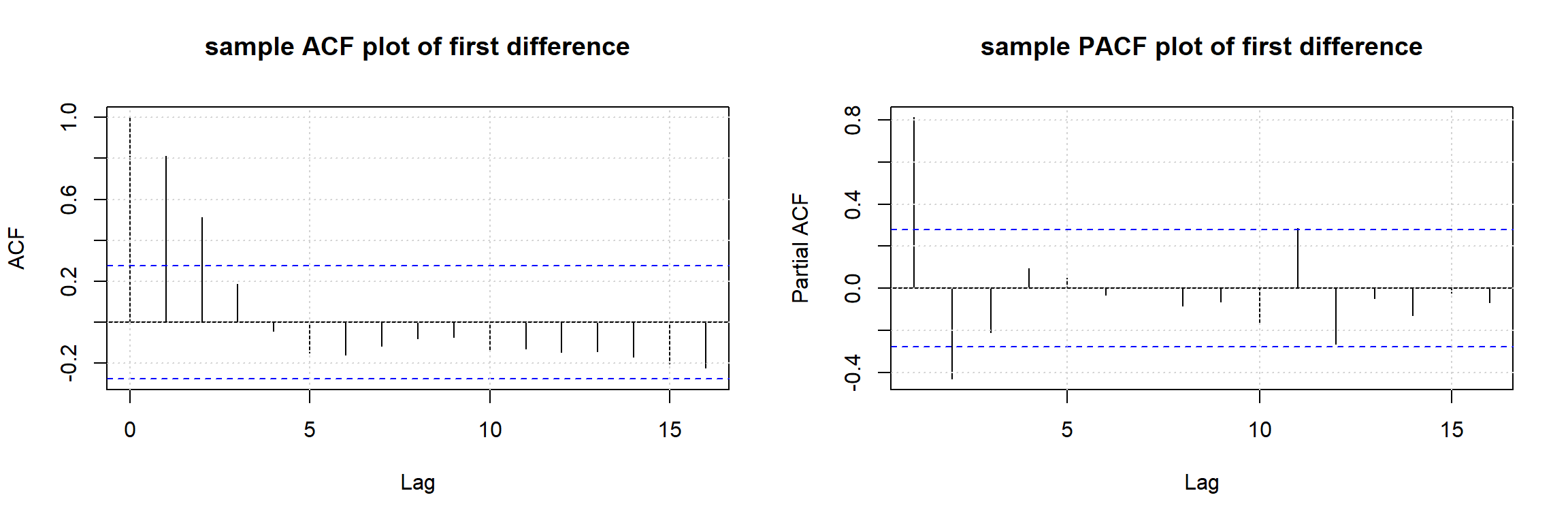## Model building and diagnostic

Let us make three ARIMA model with orders as proposed earlier. We retain last 10 observaitons for forecasting and use first 40 observations to fit the models.

## partition into train and test
train_series=timeseries[1:40]
test_series=timeseries[41:50]

## make arima models
arimaModel_1=arima(train_series, order=c(0,1,2))
arimaModel_2=arima(train_series, order=c(1,1,0))
arimaModel_3=arima(train_series, order=c(1,1,2))

## look at the parameters
print(arimaModel_1);print(arimaModel_2);print(arimaModel_3)
##
## Call:
## arima(x = train_series, order = c(0, 1, 2))
##
## Coefficients:
##          ma1    ma2
##       0.7408  1.000
## s.e.  0.2135  0.537
##
## sigma^2 estimated as 0.9673:  log likelihood = -57.78,  aic = 121.57
##
## Call:
## arima(x = train_series, order = c(1, 1, 0))
##
## Coefficients:
##          ar1
##       0.8012
## s.e.  0.0892
##
## sigma^2 estimated as 1.311:  log likelihood = -61.13,  aic = 126.26
##
## Call:
## arima(x = train_series, order = c(1, 1, 2))
##
## Coefficients:
##          ar1     ma1     ma2
##       0.5585  0.4438  0.7238
## s.e.  0.1581  0.1584  0.1305
##
## sigma^2 estimated as 0.8554:  log likelihood = -53.64,  aic = 115.29

The model parameters are shown above. R estimates the paraemters using log likelihood. The sign of $$\theta s$$ returned by R for MA part is consistent with the formula used here.

Along with model parameters, other information are also printed. From the standard errors, we see that all the parameters are more than 2 statndard deviation away from zero. So all parameters passed the t-test. The model also calculated the variance of the error term contained in the model. It also gives the likelihood and the aic values. We see that the third model is best based on likelihood and aic.

forecast1=predict(arimaModel_1, 10)
forecast2=predict(arimaModel_2, 10)
forecast3=predict(arimaModel_3, 10)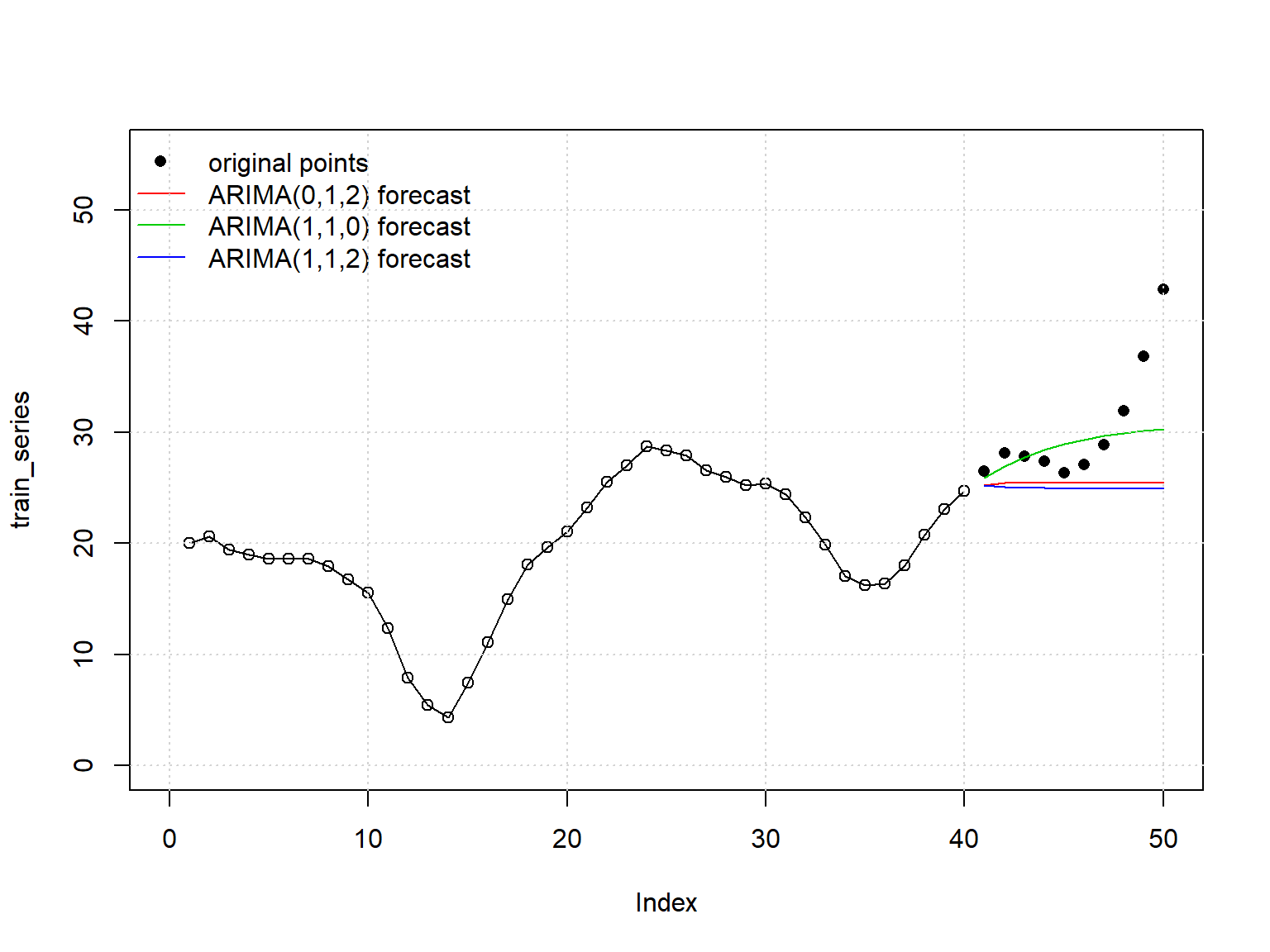From the forecst point of view, model 2, $$ARIMA(1,1,0)$$ seems to be doing a better job. In the above figure we made forecast for the next 10 time intervals. The second ARIMA model seems to stay more closely to the original observations.

In the following figure we plotted the 95% prediction intervals for the last 10 observations. The last two models generate almost similar bads. The band for the first model is narrower, despite of the fact that it is constantly underestimating. And only one, the very last observation falls outside this band.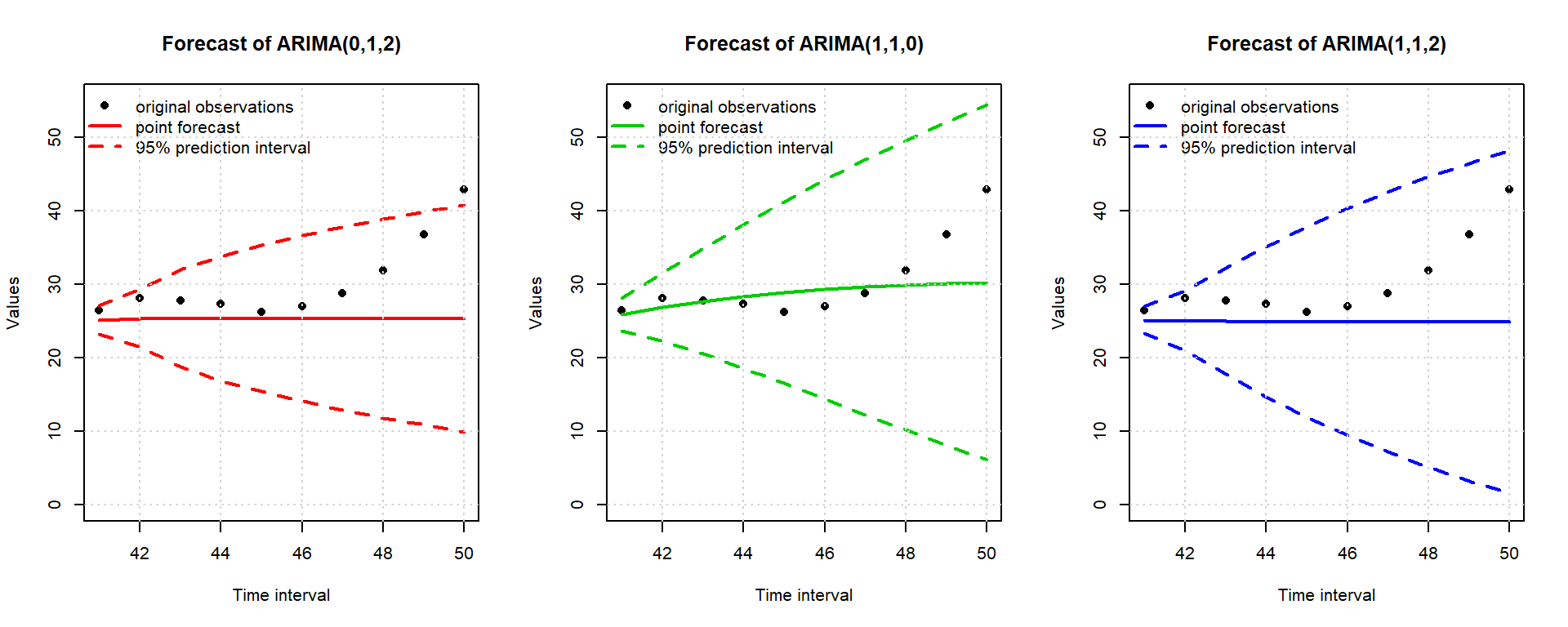Let us find the 10-step ahead forecast accuracy of the models. Below some forecast accuracy metrics are printed for the three model. We see that the second model is consistently performing better, despite not having the best AIC of likelihood value.

library(DMwR)
## Warning: package 'DMwR' was built under R version 3.4.3
accmeasures1=regr.eval(test_series, forecast1$pred) accmeasures2=regr.eval(test_series, forecast2$pred)
accmeasures3=regr.eval(test_series, forecast3\$pred)
accMeasure=rbind(accmeasures1,accmeasures2,accmeasures1)
print(accMeasure)
##                   mae      mse     rmse       mape
## accmeasures1 4.975750 51.12412 7.150113 0.14399552
## accmeasures2 2.973541 22.12474 4.703694 0.08507158
## accmeasures1 4.975750 51.12412 7.150113 0.14399552

Let us check the normality of the residuals of our three models. In the follwing figure the histograms of the residuals are shown, which does not reveal a serious deviation from normality. Next formal test (Anderson Darling test) was run to confirm the normality. The p-values are well above rejection threshold. So the residuals are not significantly different from normality.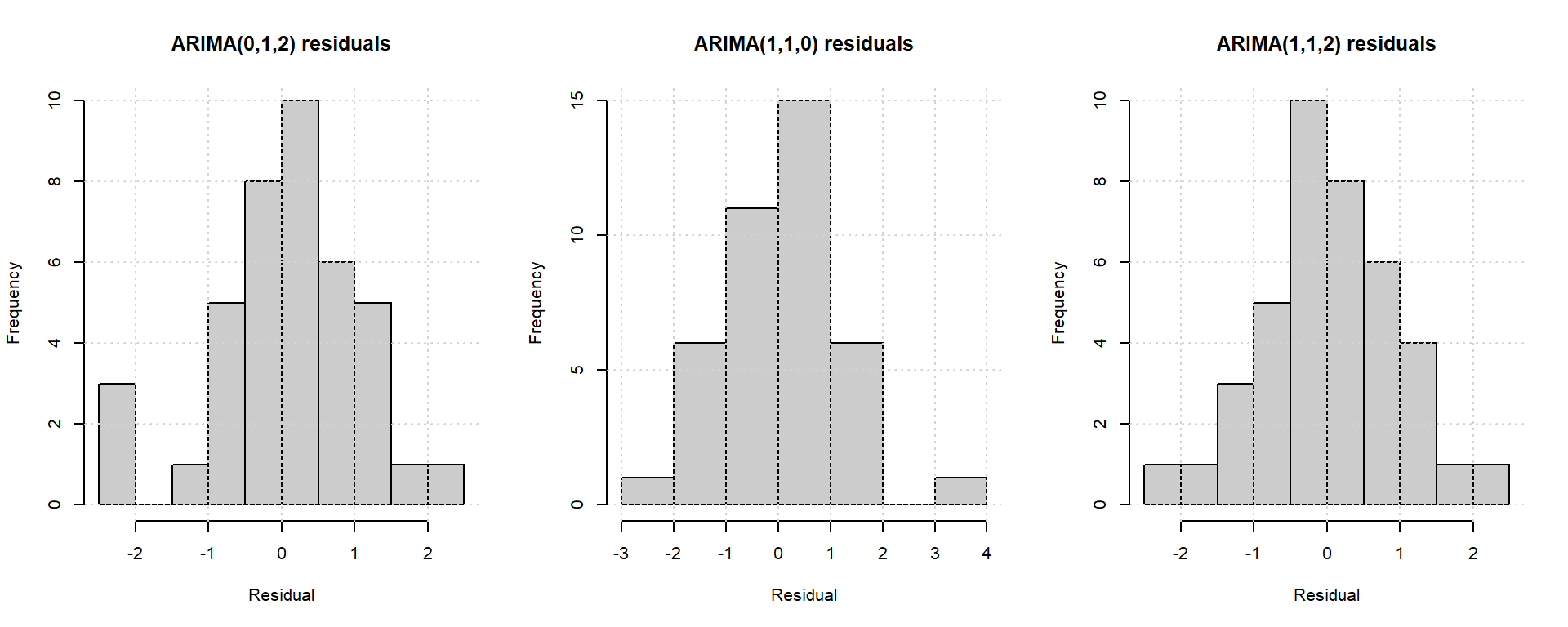##      adtest_Pvalue_Model1 adtest_Pvalue_Model2 adtest_Pvalue_Model3
## [1,]            0.3361532            0.5451065            0.9147027

## Using auto.arima function

In the preceding part we manally examined different aspects of the time series in order to find the correct specification in R. But we can do it in R using the auto.arima() function of the forecast package. Let us see what this functions gives us.

library(forecast)
## Warning: package 'forecast' was built under R version 3.4.2
AutoArimaModel=auto.arima(train_series)
AutoArimaModel
## Series: train_series
## ARIMA(1,1,2)
##
## Coefficients:
##          ar1     ma1     ma2
##       0.5585  0.4438  0.7238
## s.e.  0.1581  0.1584  0.1305
##
## sigma^2 estimated as 0.9267:  log likelihood=-53.64
## AIC=115.29   AICc=116.46   BIC=121.94

We see that the auto.arima returns us a model identical to our third proposed model. This function actually searches for a range of $$p$$, $$q$$ values, after fixing $$d$$ by Kwiatkowski-Phillips-Schmidt-Shin (KPSS) test. It chooses the model having lowest AIC score.